Test: Logic Gates & Boolean Algebra - 1

# Test: Logic Gates & Boolean Algebra - 1

Test Description

## 10 Questions MCQ Test Topicwise Question Bank for Electronics Engineering | Test: Logic Gates & Boolean Algebra - 1

Test: Logic Gates & Boolean Algebra - 1 for Electronics and Communication Engineering (ECE) 2023 is part of Topicwise Question Bank for Electronics Engineering preparation. The Test: Logic Gates & Boolean Algebra - 1 questions and answers have been prepared according to the Electronics and Communication Engineering (ECE) exam syllabus.The Test: Logic Gates & Boolean Algebra - 1 MCQs are made for Electronics and Communication Engineering (ECE) 2023 Exam. Find important definitions, questions, notes, meanings, examples, exercises, MCQs and online tests for Test: Logic Gates & Boolean Algebra - 1 below.
Solutions of Test: Logic Gates & Boolean Algebra - 1 questions in English are available as part of our Topicwise Question Bank for Electronics Engineering for Electronics and Communication Engineering (ECE) & Test: Logic Gates & Boolean Algebra - 1 solutions in Hindi for Topicwise Question Bank for Electronics Engineering course. Download more important topics, notes, lectures and mock test series for Electronics and Communication Engineering (ECE) Exam by signing up for free. Attempt Test: Logic Gates & Boolean Algebra - 1 | 10 questions in 30 minutes | Mock test for Electronics and Communication Engineering (ECE) preparation | Free important questions MCQ to study Topicwise Question Bank for Electronics Engineering for Electronics and Communication Engineering (ECE) Exam | Download free PDF with solutions
 1 Crore+ students have signed up on EduRev. Have you?
*Multiple options can be correct
Test: Logic Gates & Boolean Algebra - 1 - Question 1

### Consider a Boolean gate (D) where the output Y is related to the inputs A and B as, Y = A + B̅, where + denotes logical OR operation. The Boolean inputs ‘0’ and ‘1’ are also available separately. Using instances of only D gates and inputs ‘0’ and ‘1’, __________ (select the correct option(s)).

Detailed Solution for Test: Logic Gates & Boolean Algebra - 1 - Question 1

The given function is,
y = A + B' = (A'B)'
f(A,B) = A+B'
As 0 and 1 are available.
f(0,B) = A + B' = B'= NOT Gate.
f(A,B') = A + (B')' = A + B = OR Gate.
f(o,f(A,B')) = 0 +(A + B)' = NOR Gate.
f(A',B) = A' + B' = (A.B)' = NAND Gate.
f(0,f(A',B)) = 0+((A.B)')' = A.B = AND Gate.
With the combination of OR and NOT, NOR gate can be implemented. Since NOR gate is universal logic gate, so all the functions can be implemented.
Hence, we can implement NOT Gate we can also implement OR, AND, NOR and NAND Gate.
Hence the correct answer is option 1 and option 3.

Test: Logic Gates & Boolean Algebra - 1 - Question 2

### For the given digital circuit. NOT gate is having a time delay of 5 msec. Find for which time range the LED will glow up.  X input is logic 1 for 10 msec to 35msec as shown in the figure. (logic 1 is considered as 5 V and logic 0 as 0 V)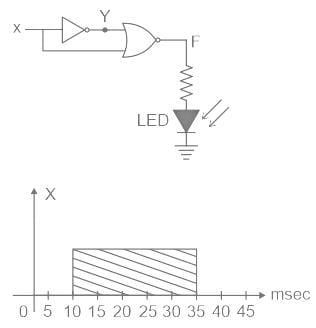Detailed Solution for Test: Logic Gates & Boolean Algebra - 1 - Question 2

output F = (X + Y)'
waveforms are given as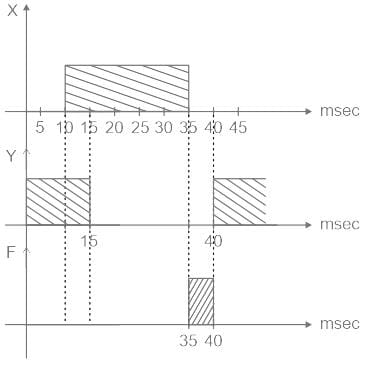As we can see for the time 35msec to 40msec output F is logic 1 (5 V)

Test: Logic Gates & Boolean Algebra - 1 - Question 3

### The Boolean equation X = [(A + B̅) (B + C)] B can be simplified to

Detailed Solution for Test: Logic Gates & Boolean Algebra - 1 - Question 3

X = [(A + B̅) (B + C)] B
= (AB + AC + 0 +  B̅C)B
= AB + ABC
= AB(1 + C)
= AB

Test: Logic Gates & Boolean Algebra - 1 - Question 4

Identify the number of prime implicants and essential prime implicants for the function
f(A, B, C, D) = Σ m(0, 1, 4, 6, 7, 8, 10, 14, 15)
(where m represents min terms)

Detailed Solution for Test: Logic Gates & Boolean Algebra - 1 - Question 4

Concept:
Prime implicant:
Each square (or) rectangle made up of the group of adjacent min terms is called a sub cube. Each of these sub cubes is called a prime implicant.
Essential prime implicant: The prime implicant which contains at least one ‘1’ which can not be covered by any other prime implicant is called essential prime implicant.
Redundant prime implicant: The prime implicant whose each ‘1’ is covered least by one essential prime implicant is called a redundant prime implicant.

Application:
Given Boolean function is: f(A, B, C, D) = Σ m(0, 1, 4, 6, 7, 8, 10, 14, 15)
The K-map is as shown below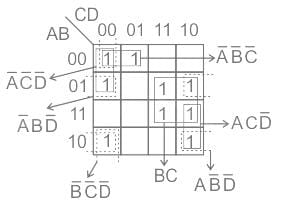From the above K-map,
The prime implicants: BC, A̅ B̅ C̅, ACD̅, AB̅ D̅, B̅ C̅ D̅, A̅ BD̅, A̅ C̅ D̅
The essential prime implicants: BC, A̅ B̅ C̅

Test: Logic Gates & Boolean Algebra - 1 - Question 5

Find the output Boolean function for the logic circuit.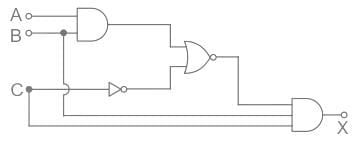Detailed Solution for Test: Logic Gates & Boolean Algebra - 1 - Question 5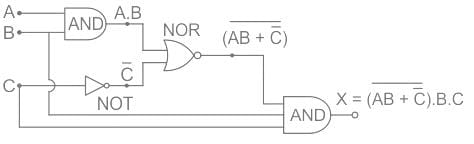After simplification, we get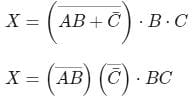∵ De Morgan’s law: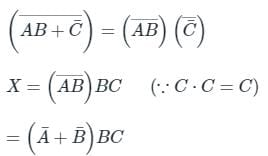∵ De Morgan’s law: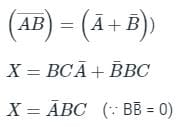Test: Logic Gates & Boolean Algebra - 1 - Question 6

If the Boolean expression P̅Q + QR + PR is minimized, the expression becomes:

Detailed Solution for Test: Logic Gates & Boolean Algebra - 1 - Question 6

Concept:
Consensus Law is one of the most powerful theorems used in digital electronics for the minimization of Boolean function or equation either in the successive reduction method or in the K-Map method.
Statement:

• The consensus theorem states that the consensus term of a disjunction is defined when the terms in function are reciprocals to each other (such as A and A̅).
• The consensus theorem is defined in two statements (normal form and it's dual). They are
• AB + ĀC+BC = AB+ĀC
• (A+B)(Ā+C)(B+C) = (A+B)( Ā+C)

Calculation:
Y = P̅Q + QR + PR
Y = P̅Q + PR + QR (P̅ + P)
Y = P̅Q + PR + QRP̅ + QRP
Y = P̅Q(1 + R) + PR(1 + Q)
Y = P̅Q + PR where (1 + A = 1) according to Boolean algebra.

Test: Logic Gates & Boolean Algebra - 1 - Question 7

The output of logic circuit given below represents _______ gate.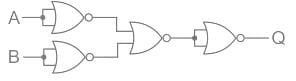Detailed Solution for Test: Logic Gates & Boolean Algebra - 1 - Question 7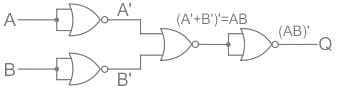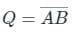Output expression Q is equivalent to NAND gate.

Test: Logic Gates & Boolean Algebra - 1 - Question 8

Simplify the following expression.
Y = AB + A(B + C) + B(B + C)

Detailed Solution for Test: Logic Gates & Boolean Algebra - 1 - Question 8

Analysis:
Y = AB + A(B + C) + B(B + C)
= AB + AB + AC + B + BC
Since AB + AB = AB, we get:
Y = AB + AC + B (1 + C)
Since 1 + X (any variable) = X, we get:
Y = AB + AC + B
Y = B(1 + A) + AC
Y = B + AC

Test: Logic Gates & Boolean Algebra - 1 - Question 9

The Boolean expression AB + AC̅ + BC simplifies to

Detailed Solution for Test: Logic Gates & Boolean Algebra - 1 - Question 9

Concept:
3 variable K-maps:

• For a 3-variable Boolean function, there is a possibility of 8 output minterms.
• The general representation of all the minterms using 3-variables is shown below.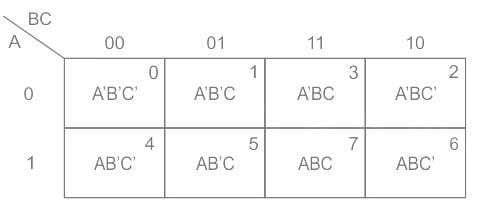Calculation:
Given Boolean expression is,
F = AB + AC̅ + BC
= A B C̅ + A B C + A B̅ C̅ + A B C̅ + A B C + A̅ B C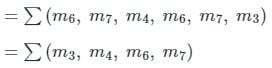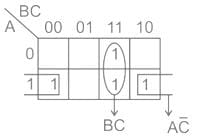F = BC + AC̅

Test: Logic Gates & Boolean Algebra - 1 - Question 10

The minterm expansion of f (P, Q, R) = PQ + QR̅ + PR̅ is

Detailed Solution for Test: Logic Gates & Boolean Algebra - 1 - Question 10

F(P, Q, R) = PQ + QR' + PR'
= PQ (R + R') + (P + P')QR' + P(Q + Q')R'
= PQR + PQR' + PQR' + P'QR' + PQR' + PQ'R'
= PQR + PQR' + P'QR' + PQ'R'
= m7 + m6 + m2 + m4
= m2 + m4 + m6 + m7

## Topicwise Question Bank for Electronics Engineering

193 tests
Information about Test: Logic Gates & Boolean Algebra - 1 Page
In this test you can find the Exam questions for Test: Logic Gates & Boolean Algebra - 1 solved & explained in the simplest way possible. Besides giving Questions and answers for Test: Logic Gates & Boolean Algebra - 1, EduRev gives you an ample number of Online tests for practice

## Topicwise Question Bank for Electronics Engineering

193 tests(Scan QR code)